# Freudenthal-Kantor triple system

A triple system considered for constructing all simple Lie algebras (cf. Lie algebra), and introduced as an algebraic system which is a generalization both of the algebraic systems appearing in the metasymplectic geometry developed by H. Freudenthal and of a generalized Jordan triple system of second order developed by I.L. Kantor.

Recall that a triple system is a vector spaceover a fieldtogether with a-trilinear mapping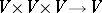.

For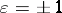, a vector space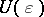over a fieldwith the trilinear productis called a Freudenthal–Kantor triple system if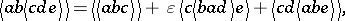(a1)(a2)

where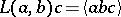and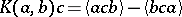.

In particular, a Freudenthal–Kantor triple systemis said to be balanced if there exists a bilinear formsuch that, for all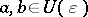.

This balancing property is closely related to metasymplectic geometry.

Note that if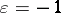and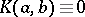(identically), then the Freudenthal–Kantor triple system reduces to a Jordan triple system.

As the notion of a Freudenthal–Kantor triple system includes the notions of a generalized Jordan triple system of second order, a structurable algebra, and an Allison–Hein triple system, it is useful in obtaining all Lie algebras, without the use of root systems and Cartan matrices.

Letbe a vector space with a bilinear form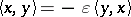. Thenis a Freudenthal–Kantor triple system with respect to the triple product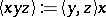. In particular, it is important that the linear span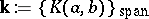of the set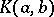makes a Jordan triple system of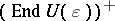with respect to the triple product.

Letbe a Freudenthal–Kantor triple system. The vector space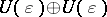becomes a Lie triple system with respect to the triple product defined by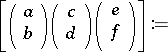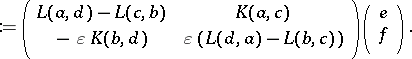Using this, one can obtain the Lie triple systemassociated with; it is denoted be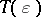.

Using the concept of the standard embedding Lie algebra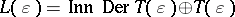associated with a Lie triple system, one can obtain the construction ofassociated with a Freudenthal–Kantor triple system. In fact, putequal to the linear span of the endomorphisms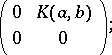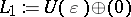;;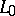equal to the linear span of the endomorphisms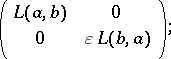equal to the linear span of the endomorphisms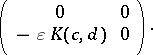Then one obtains the decomposition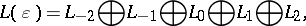and, more precisely,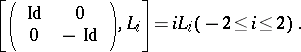These results imply the dimensional formula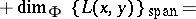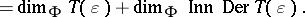This algebra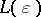is called the Lie algebra associated with.

The concepts of a triple system and a supertriple system are important in the theory of quarks and Yang–Baxter equations.

Note that a "triple system" in the sense discussed above is totally different from "triple system" in combinatorics (see, e.g., Steiner triple system).